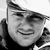# QlikView App Dev

Discussion Board for collaboration related to QlikView App Development.

Announcements
Now Live: Qlik Sense SaaS Simplified Authoring – Analytics Creation for Everyone: READ DETAILS
cancel
Showing results for
Did you mean:Contributor II

## Variable greater than or less than variable

I have a pivot table with a single expression. The requirement is to allow the user to filter the data in the pivot table based on two variables.

Variable 1: will be less than or greater than ( > or <)

Variable2: some integer

I would like to be able to have the user select < or > and enter a value and have the pivot table only show lines that fall within the variable range.

My current expression doesn't respond at all. =if(sum( Planned) - sum( Actual) & \$(Variable1) & \$(Variable2),
sum(Planned) - sum(Actual),null())

Any guidance would be appreciated.

1 Solution

Accepted SolutionsMVP

Try removing the & signs like this :

=if(sum(Planned) - sum( Actual)  \$(Variable1) \$(Variable2),sum(Planned) - sum(Actual),null())

2 RepliesMVP

Try removing the & signs like this :

=if(sum(Planned) - sum( Actual)  \$(Variable1) \$(Variable2),sum(Planned) - sum(Actual),null())Contributor II
Author

That worked! Thanks. How come when I use that expression the syntax checker doesn't accept it but it still works?